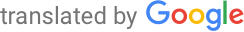Machine-translated page for increased accessibility for English questioners.

# Image processing

1. Image data acquisition. Radiation sources and detectors, cameras and their properties, types of noise. Image encoding, transmission and storage. Image formation in optical systems, optical resolution, PSF, optical defects, microscopes and telescopes. Detection of multidimensional image data (3D, spectral, time series). Automation of image information acquisition.
`PA172`
2. Digital filters. Histogram analysis methods, edge detection, discrete transformations (Fourier, wavelet), Hough / Radon transform, recursive filtering, deconvolution, image descriptors, image compression, resampling, linear and nonlinear filters.
`PA171`
3. Digital geometry. Grids, digitization, adjacency, incidence, marking of components, estimation of geometric and topological properties of digital sets, digital metrics (Euclidean and geodetic), approximation of Euclidean metrics, calculation of distance map, Freeman code, description of objects, skeletons.
`PA170`
4. Mathematical morphology. Properties of morphological operators (arrangement, idempotence, etc.). Dilation and erosion. Top hat. Morphological and algebraic openings and closures. Granulometry. Hit-or-miss transformation. Geodetic transformations and morphological reconstruction. Morphological filters. Segmentation using mathematical morphology.
`PA173`
5. Image analysis. Diffusion filtering, variational filtering, image segmentation. Level set methods, active curves and surfaces (geodetic model, Chan-Vese model). Optical flow, image registration, minimization using graph sections, classification of objects.
`PA166/PV131`
6. Geometric algorithms. Convex packaging, construction in 2D and 3D. Packaging bodies, packaging hierarchy, packaging efficiency. Voronoi diagrams, Delaunay triangulation, duality, spatial search (data structures, algorithms).
`M7130/PA010`
7. Graphs and graph algorithms. Formalization of basic graph concepts, representation of graphs. Coherence of a graph, chromaticity, planar graphs. Algorithms (including the complexity and basic idea of proofs of correctness): searching the graph in width and depth, the shortest distances, skeletons, flows in networks.
`MA010, MA015`
8. Statistics. Descriptive statistics. Discrete and continuous random variables (NV), basic distribution. Numerical characteristics of NV. Central limit theorem. Point estimates, confidence intervals, testing of statistical hypotheses, level of significance. Basic parametric and nonparametric tests, ANOVA, NV independence tests. Linear regression, total F-test, partial t-tests.
`MV011`
9. Algorithms and data structures. Complexity analysis, amortized complexity. Algorithm design techniques (divide and conquer, dynamic programming, hunger strategies). Advanced data structures (heaps, union-find structures). Algorithms for working with strings (Karp-Rabin, KMP, Boyer-Moore algorithms, use of finite automata).
`IV003`
10. Powerful computers and intensive calculations. Superscalar and streaming (GPU) processors, intraprocessor and internuclear parallelism. Memory organization, shared and distributed, cache coherence. Code optimization. Distributed systems, decomposition of tasks, basic programming support.
`PA039`